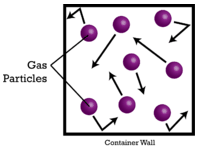# 10.13: Avogadro's Hypothesis and Molar Volume

Knowing the amount of gas available for a dive is crucial to a diver's survival. The tank on the diver's back is equipped with gauges to indicate how much gas is present and what the pressure is. A basic knowledge of gas behavior allows the diver to assess how long they can stay underwater without developing problems.

## Avogadro's Hypothesis and Molar Volume

Volume is a third way to measure the amount of matter, after item count and mass. With liquids and solids, volume varies greatly depending on the density of the substance. This is because solid and liquid particles are packed close together with very little space in between the particles. However, gases are largely composed of empty spaces between the actual gas particles (see figure below).Figure $$\PageIndex{1}$$: Gas particles are very small compared to the large amounts of empty space between them.

In 1811, Amadeo Avogadro explained that the volumes of all gases can be easily determined. Avogadro's hypothesis states that equal volumes of all gases at the same temperature and pressure contain equal numbers of particles. Since the total volume that a gas occupies is made up primarily of the empty space between the particles, the actual size of the particles themselves is nearly negligible. A given volume of a gas with small light particles, such as hydrogen $$\left( \ce{H_2} \right)$$, contains the same number of particles as the same volume of a heavy gas with large particles, such as sulfur hexafluoride, $$\ce{SF_6}$$.

Gases are compressible, meaning that when put under high pressure, the particles are forced closer to one another. This decreases the amount of empty space and reduces the volume of the gas. Gas volume is also affected by temperature. When a gas is heated, its molecules move faster and the gas expands. Because of the variation in gas volume due to pressure and temperature changes, the comparison of gas volumes must be done at standard temperature and pressure. Standard temperature and pressure (STP) is defined as $$0^\text{o} \text{C}$$ $$\left( 273.15 \: \text{K} \right)$$ and $$1 \: \text{atm}$$ pressure. The molar volume of a gas is the volume of one mole of a gas at STP. At STP, one mole ($$6.02 \times 10^{23}$$ representative particles) of any gas occupies a volume of $$22.4 \: \text{L}$$ (figure below).Figure $$\PageIndex{2}$$: A mole of any gas occupies $$22.4 \: \text{L}$$ at standard temperature and pressure ($$0^\text{o} \text{C}$$ and $$1 \: \text{atm}$$).

The figure below illustrates how molar volume can be seen when comparing different gases. Samples of helium $$\left( \ce{He} \right)$$, nitrogen $$\left( \ce{N_2} \right)$$, and methane $$\left( \ce{CH_4} \right)$$ are at STP. Each contains 1 mole or $$6.02 \times 10^{23}$$ particles. However, the mass of each gas is different and corresponds to the molar mass of that gas: $$4.00 \: \text{g/mol}$$ for $$\ce{He}$$, $$28.0 \: \text{g/mol}$$ for $$\ce{N_2}$$, and $$16.0 \: \text{g/mol}$$ for $$\ce{CH_4}$$.Figure $$\PageIndex{3}$$: Avogadro's hypothesis states that equal volumes of any gas at the same temperature and pressure contain the same number of particles. At standard temperature and pressure, 1 mole of any gas occupies $$22.4 \: \text{L}$$.

## Summary

• Equal volumes of gases under the same conditions contain the same number of particles, according to Avogadro's hypothesis.
• Standard temperature and pressure is defined as $$0^\text{o} \text{C}$$ $$\left( 273.15 \: \text{K} \right)$$ and $$1 \: \text{atm}$$ pressure.